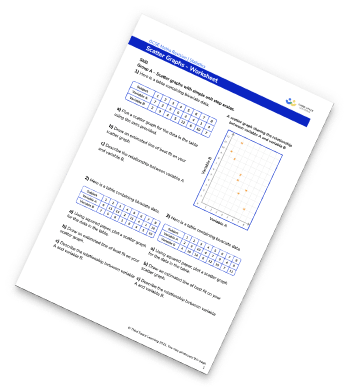# GCSE Maths Worksheets

Free topic specific maths worksheets to help your students prepare for their maths GCSE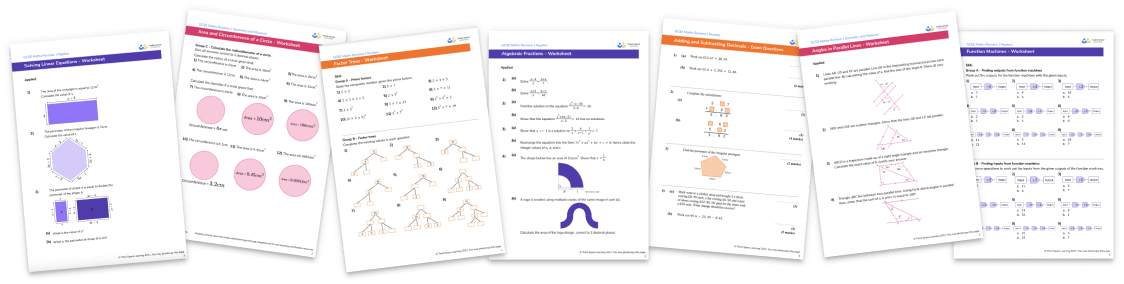Algebra worksheets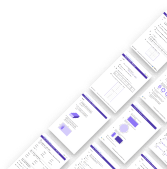Number worksheets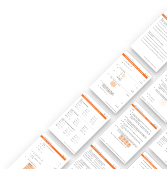Geometry and measure worksheets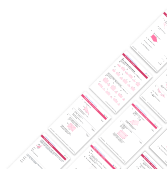Ratio and proportion worksheets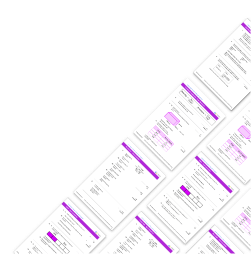Probability worksheets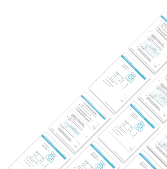Statistics worksheets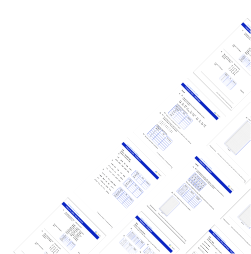## Do you have KS4 students who need more focused attention to succeed at GCSE?There will be students in your class who require individual attention to help them succeed in their maths GCSEs. In a class of 30, it’s not always easy to provide.

Help your students feel confident with exam-style questions and the strategies they’ll need to answer them correctly with our dedicated GCSE maths revision programme.

Lessons are selected to provide support where each student needs it most, and specially-trained GCSE maths tutors adapt the pitch and pace of each lesson. This ensures a personalised revision programme that raises grades and boosts confidence.

Find out more

## Popular algebra worksheets

Simultaneous Equations Worksheet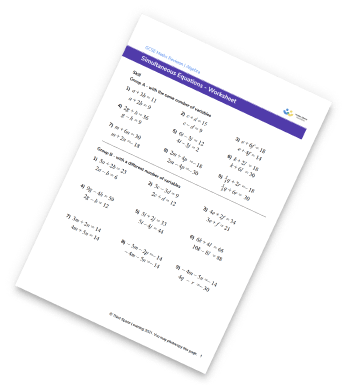Factorising Quadratics Worksheet (Double Brackets)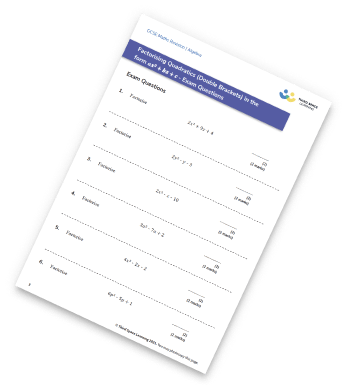Algebraic Fractions Worksheet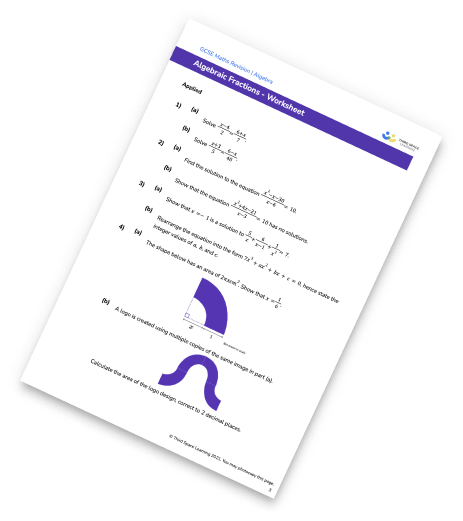Nth Term Worksheet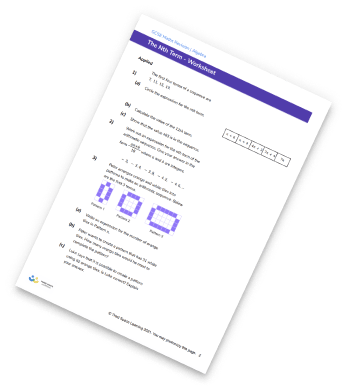## Popular number worksheets

Equivalent Fractions Worksheet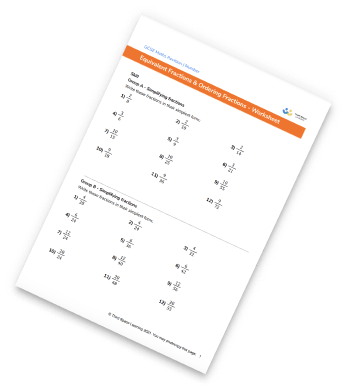Percentage Increase And Decrease Worksheet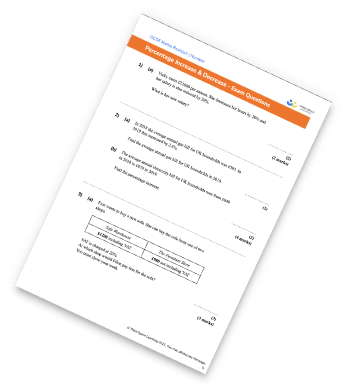Negative Numbers Worksheet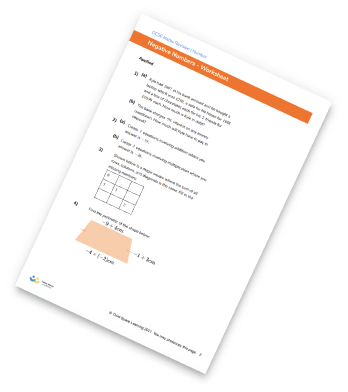Adding And Subtracting Decimals Worksheets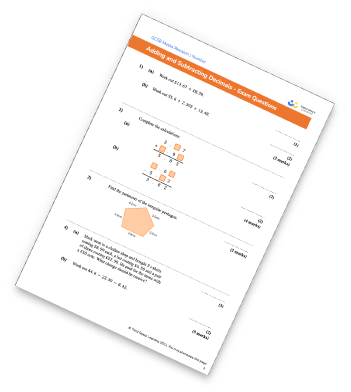## Popular geometry and measure worksheets

Area of a Triangle (1/2abSinC) Worksheet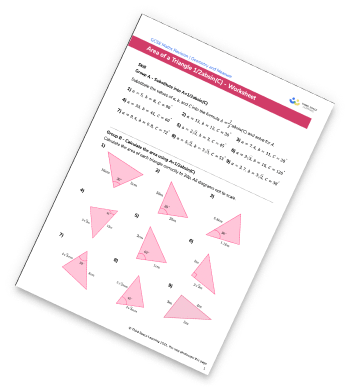Angles In Polygons Worksheet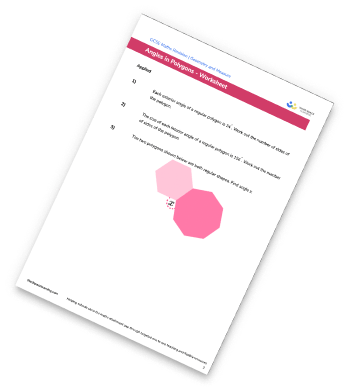Area and Circumference of a Circle Worksheet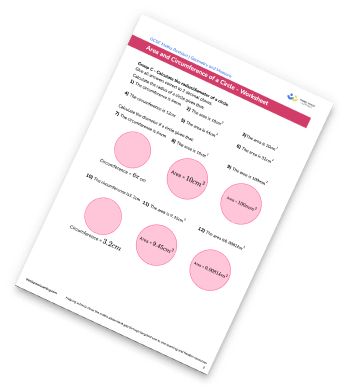Trigonometry Worksheet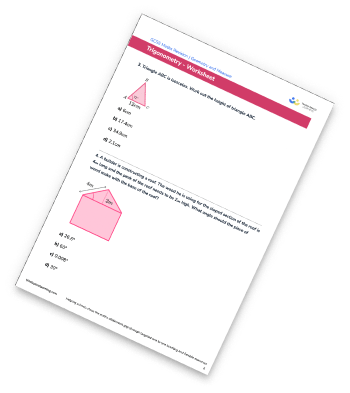## Popular ratio and proportion worksheets

Direct Proportion Worksheet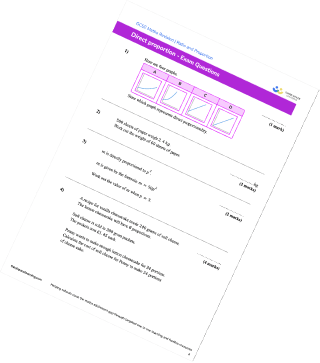Proportion Worksheet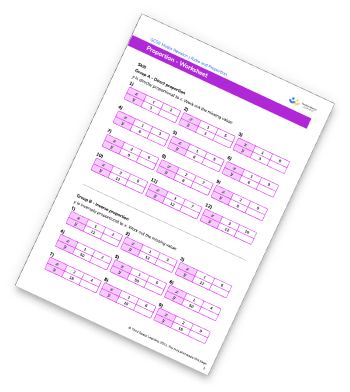Speed Distance Time Worksheet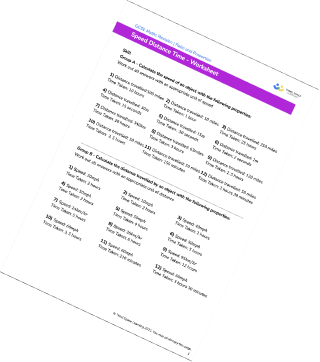Compound Measures Worksheet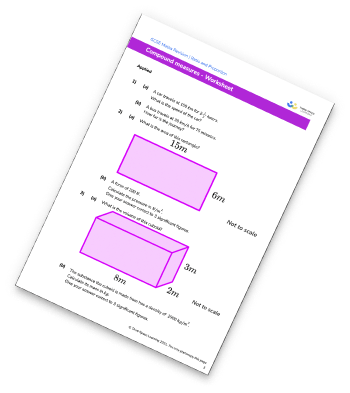## Popular probability worksheets

Venn Diagram Worksheet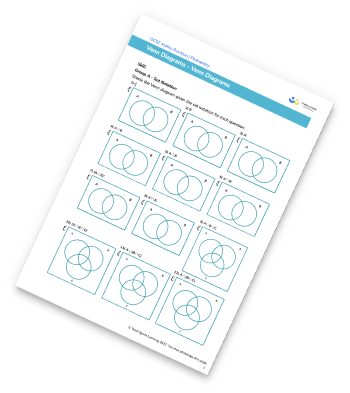Probability Worksheet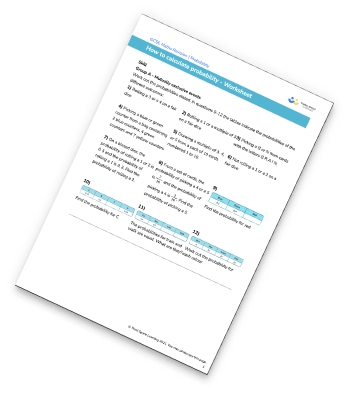Probability Scale Worksheet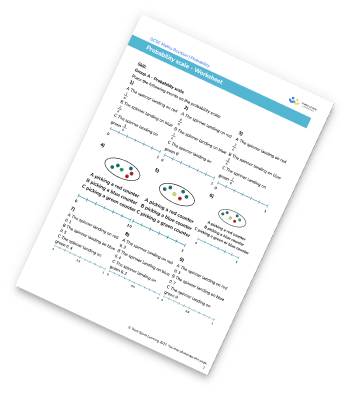Sample Space Worksheet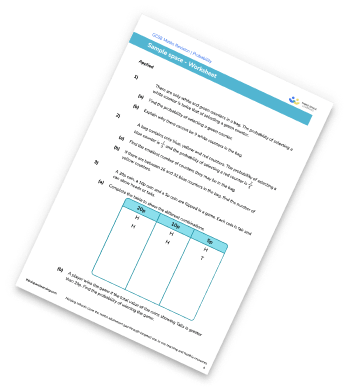## Popular statistics worksheets

Mean, Median, Mode, and Range Worksheet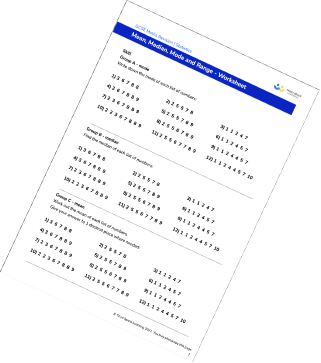Frequency Table Worksheet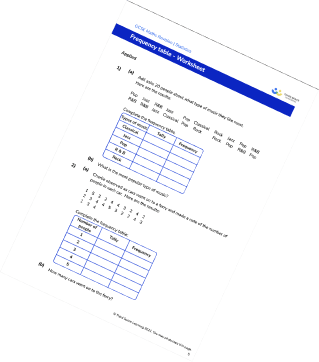Bar Chart Worksheets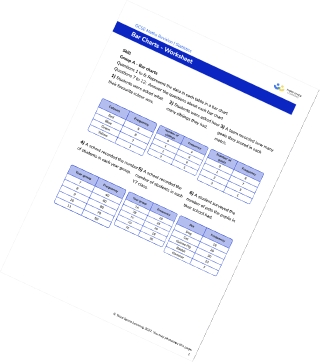Scatter Graphs Worksheet